# Kraken Daily Market Report for March 28 2021

## Overview

• Total spot trading volume at $840.4 million, down from the 30-day average of$1.41 billion.
• Total futures notional at $373.1 million. • The top five traded coins were, respectively, Bitcoin, Tether, Ethereum, StorJ, and Cardano. • Strong returns from Decentraland Mana (+23%) and The Graph (+23%). March 28, 2021$840.4M traded across all markets today
Crypto, EUR, USD, JPY, CAD, GBP, CHF, AUD
XBT
$55766. ↓0.22%$364.0M
USDT
$0.9997 ↓0.04%$109.6M
ETH
$1685.3 ↓1.8%$98.3M
STORJ
$2.7349 ↓15%$41.0M
$1.1894 ↑0.8%$29.8M
DOT
$32.242 ↓1.3%$26.7M
FIL
$125.10 ↓5.3%$25.2M
MANA
$1.1391 ↑23%$23.3M
SC
$0.0247 ↑5.5%$21.6M
USDC
$0.9999 ↑0.0%$21.2M
XRP
$0.5459 ↓0.5%$12.2M
KSM
$512.18 ↑9.0%$11.4M
XLM
$0.3960 ↑3.3%$7.3M
$26.646 ↓1.0%$6.85M
ALGO
$1.2343 ↑6.1%$6.65M
CRV
$3.0180 ↓4.4%$6.62M
GRT
$1.8117 ↑23%$6.58M
LTC
$184.18 ↓0.11%$6.31M
ICX
$2.3353 ↑6.5%$5.67M
FLOW
$29.730 ↑7.9%$4.57M
UNI
$27.852 ↓1.3%$4.52M
XMR
$232.17 ↑4.7%$4.34M
LSK
$4.8591 ↑7.6%$4.13M
BCH
$496.84 ↓1.0%$4.08M
ATOM
$19.282 ↑2.9%$3.33M
XDG
$0.0536 ↓1.5%$2.88M
BAT
$1.1161 ↑2.4%$2.86M
OMG
$5.8739 ↑10%$2.85M
XTZ
$4.2225 ↑2.6%$2.67M
OCEAN
$1.4374 ↑4.5%$2.54M
ZEC
$144.39 ↑7.9%$2.32M
TRX
$0.0634 ↓0.26%$2.3M
EOS
$4.1100 ↑0.1%$2.14M
OXT
$0.6743 ↑0.25%$1.9M
QTUM
$8.2077 ↓4.3%$1.82M
NANO
$4.5165 ↑1.6%$1.67M
REPV2
$35.863 ↑7.0%$1.59M
KAVA
$5.5640 ↓5.5%$1.49M
AAVE
$347.99 ↓1.1%$1.34M
KEEP
$0.6175 ↑5.9%$1.3M
MLN
$82.104 ↓5.3%$1.25M
SNX
$16.424 ↓4.5%$1.16M
WAVES
$11.780 ↓0.9%$1.09M
ANT
$9.2652 ↓7.6%$1.08M
REP
$35.667 ↑8.8%$1.04M
DASH
$207.10 ↑2.5%$871K
DAI
$1.0006 ↓0.06%$860K
EWT
$15.869 ↑3.6%$790K
YFI
$32594. ↓0.6%$751K
KNC
$2.6523 ↑7.2%$727K
PAXG
$1747.8 ↑0.6%$724K
COMP
$361.74 ↓0.6%$496K
ETC
$11.855 ↑0.3%$261K
BAL
$50.879 ↓2.4%$241K
GNO
$140.79 ↓0.6%$92.7K
TBTC
$57722. ↑1.7%$76.5K

#####################. Trading Volume by Asset. ##########################################

The figures below break down the trading volume of the largest, mid-size, and smallest assets. Cryptos are in purple, fiats are in blue. For each asset, the chart contains the daily trading volume in USD, and the percentage of the total trading volume. The percentages for fiats and cryptos are treated separately, so that they both add up to 100%.

Figure 1: Largest trading assets: trading volume (measured in USD) and its percentage of the total trading volume (March 28 2021)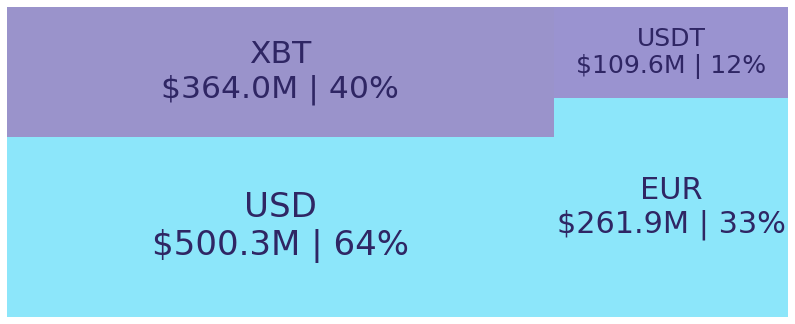Figure 2: Mid-size trading assets: (measured in USD) (March 28 2021)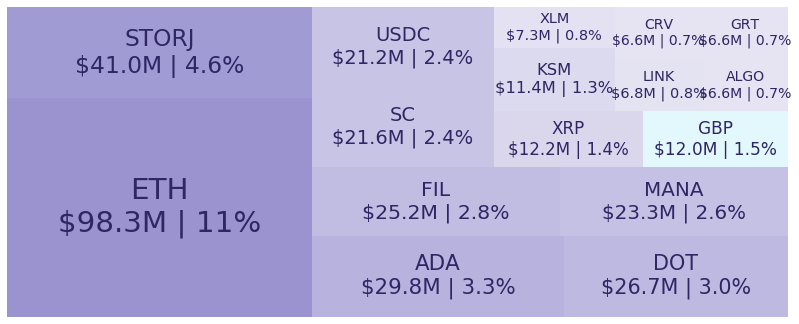Figure 3: Smallest trading assets: (measured in USD) (March 28 2021)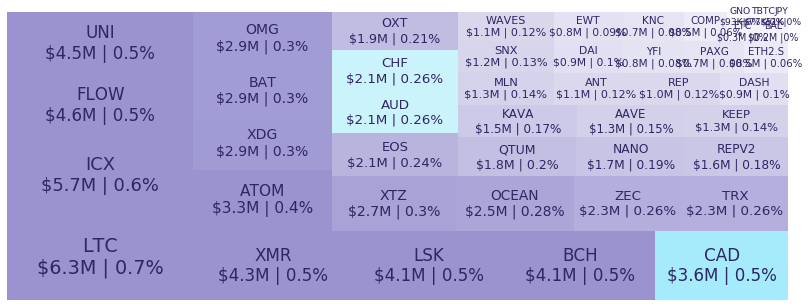Spread percentage is the width of the bid/ask spread divided by the bid/ask midpoint. The values are generated by taking the median spread percentage over each minute, then the average of the medians over the day.

Figure 4: Average spread % by pair (March 28 2021)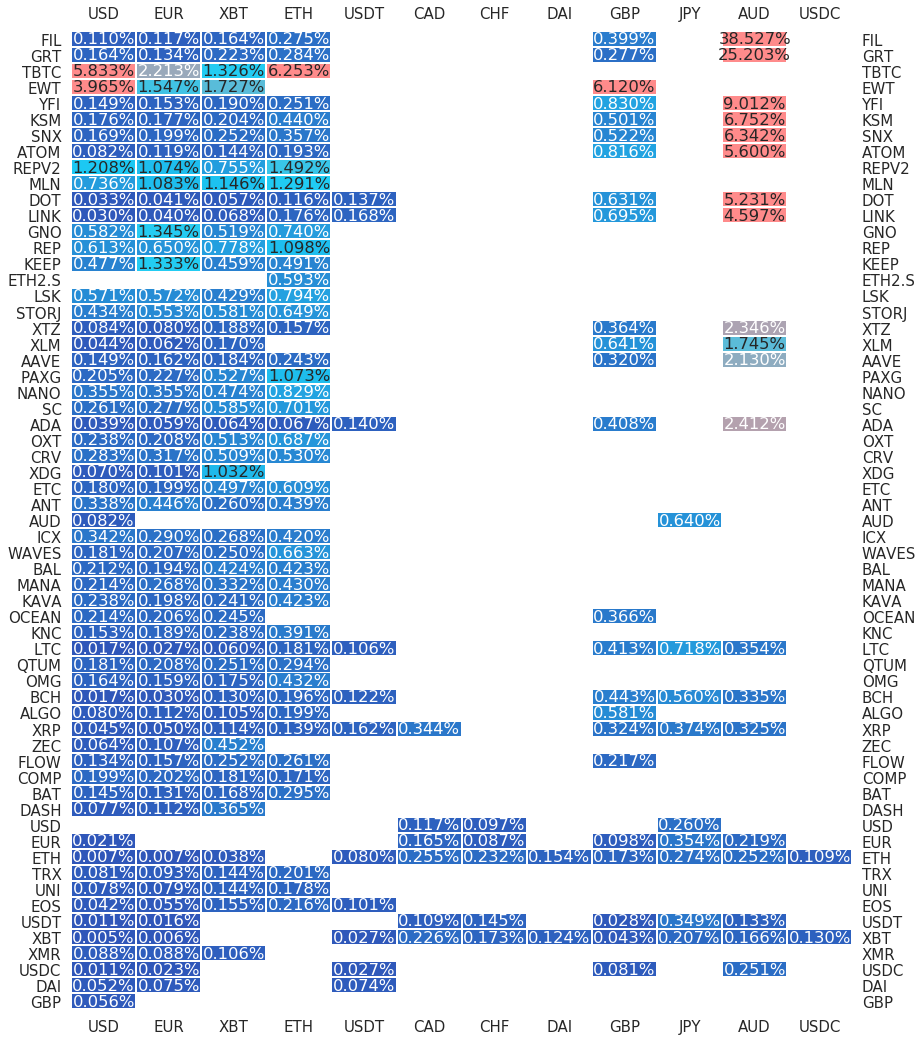.

#########. Returns and Volume ############################################

## Returns and Volume

Figure 5: Returns of the four highest volume pairs (March 28 2021)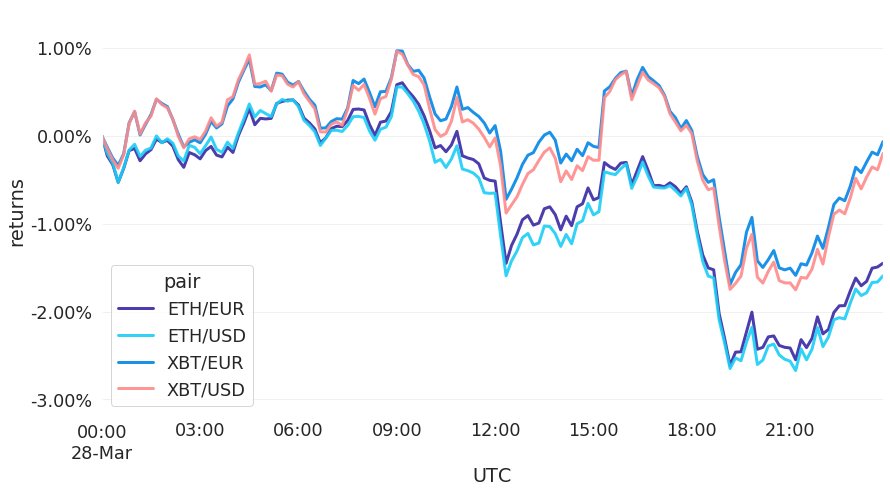Figure 6: Volume of the major currencies and an average line that fits the data to a sinusoidal curve to show the daily volume highs and lows (March 28 2021)